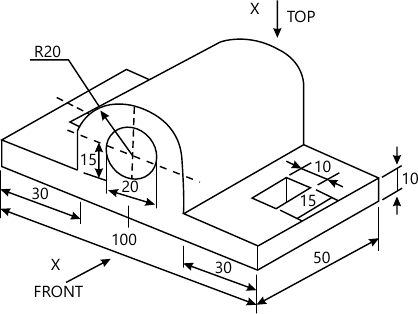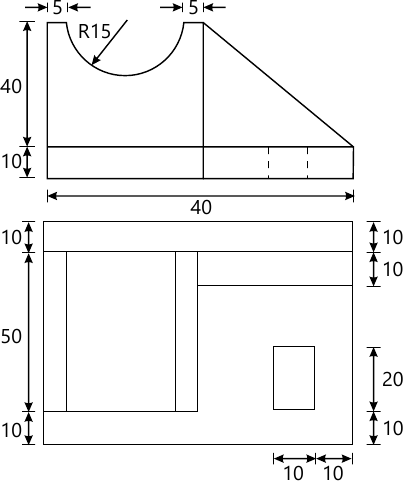MORE IN Engineering Graphics
GTU First Year Engineering (Semester 1)
Engineering Graphics
June 2015
Total marks: --
Total time: --
INSTRUCTIONS
(1) Assume appropriate data and state your reasons
(2) Marks are given to the right of every question
(3) Draw neat diagrams wherever necessary

Objective Question Attempt all questions
1 (a) 1 When the plane cuts the cone parallel to one of the generator, the curve trace out by section is
(a) Ellipse (b) Parabola (c) Hyperbola (d) Involute
1 M
1 (a) 2 In first angle projection method The Left hand side view is placed on
(a) Above elevation (b) Right side of elevation (
C) below elevation (d) Left side of elevation
1 M
1 (a) 3 A French curve is used to draw
(a) Circles
(b)Smooth freeform curve (c) Right circular cone (d) orthographic projection
1 M
1 (a) 4 If line is inclined to vertical plane and parallel to Horizontal plane, then which one of the following is always correct?
(a) True length = Plan length
(b)True length = Elevation length
(c)True length ≠ Plan length
(d)None of the above
1 M
1 (a) 5 To obtain the true shape of the section of solid, an auxiliary plane is set at
(a) Parallel to cutting plane
(b)Perpendicular to cutting plane (c ) Parallel to Ground plane
(d)Perpendicular to XY Plane
1 M
1 (a) 6 A square plane is inclined to HP & perpendicular to VP its elevation appears as
(a) Rhombus
(b)Square (c) Straight line
(d)Rectangle
1 M
1 (a) 7 Length (L) of line in Isometric view will be equal to
(a) 0.707 L
(b)0.815 L (c) True length L (d) 0.866 L
1 M
Attempt all questions
1 (b) 1 For scale, which one is not correct
(a) 1:2
(b)1:20
(c)1:1/2
(d)1/2
1 M
1 (b) 2 In Isometric view length, Width, and Height are inclined at
(a) 30°
(b)60°
(c)90°
(d)120°
1 M
1 (b) 3 When a point is below HP & in Front of VP it is in
1 M
1 (b) 4 In orthographic view the lines Perpendicular to arrow X are drawn as (1) (1) Parallel to XY in Plan (2) Parallel to XY in elevation (3) Perpendicular to XY in Elevation
(a) 1
(b)2
(c)3
(d)1&2
1 M
1 (b) 5 When the cone, resting on base on V.P., is cut by section plane parallel to V.P. then the true shape is __________ and can be seen in __________ view.
(a) Circle, Front
(b)Ellipse, Front
(c)Ellipse, Top
(d)Circle, Top.
1 M
1 (b) 6 The isometric view of a vertical line is represented at an angle of ____ in front view and having a length _________ the original length of line.
(a) 30° , Same as
(b)30° , Less than
(c)90°, Same as
(d)90° , Less than
1 M
1 (b) 7 While drawing the isometric view of the sphere, its diameter is taken as
(a) Equal to actual diameter
(b)11/9 times of the actual diameter
(c)21/9 times of the actual diameter
(d)none of the above
1 M

2 (a) Construct the ellipse if the distance between the focus and the directrix is 50 mm. & The eccentricity is 2/3. Draw the tangent and the normal to the ellipse at given point.
7 M
2 (b) The projectors of the ends of a line AB are 50mm apart. The end A is 20mm above the H.P. and 30mm in front of the V.P. The end B is 10mm below the H.P. and 40mm behind the V.P. Determine the true length of line AB, its inclinations with H.P. and V.P. and apparent angles also
7 M

3 (a) Construct the Involute of circle of 30 mm diameter for one turn. Draw tangent and normal to the Involute at any point on it..
7 M
3 (b) A line AB, 100 mm long, is inclined at 45° to HP. The end A is 10 mm above the HP and is 65 mm in front of the VP Draw projections of the line if its Front View measures 90 mm and find the inclination of the line with the VP.
7 M

4 (a) A hexagonal pyramid, side of the base 25 mm long and height 70 mm resting on HP on its side, has one of its triangular faces perpendicular to the HP and inclined at 60° to VP. Draw its projections.
7 M
4 (b) Draw an Archimedean spiral of 1.5 convolutions, the greatest and least radii being 115 mm and 25 mm respectively. Draw tangent and normal to the spiral at any point on the curve.
7 M

5 (a) A hexagonal Prism, side of base 30 mm and height 60 mm, is standing upright with base on H.P. one side of the base and axis are parallel to V.P. It is cut by section plane making an angle of 60° to H.P. and crossing the axis 10 mm from the top. Draw top view, sectional front view, sectional left hand side view and true shape of section.
7 M
5 (b) A rhombus of negligible thickness is having its diagonals 100 mm and 50 mm long. Draw the projections of the rhombus when the longer diagonal is inclined at 30° to the Horizontal Plane and 30° to Vertical Plane.
7 M

6 (a) 1, In orthographic projection why second and fourth angle projection method are not used?
2. Draw the symbol of First angle projection method.
3. Give the dimension of Title block and list the information given in it.
7 M
6 (b) Using the Third angle projection method, draw the following view for the FIGURE-1. Give the dimensions using the Aligned dimensioning method. (i) front view (ii) Top view (iii) Full Sectional Right Hand Side End View.7 M

7 (a) Construct a diagonal scale of representative fraction = (1/36) showing yard, foot and inch. Scale should be long enough to measure 5 yard.
7 M
7 (b) The orthographic views of an object using the first angle projection method are shown in the FIGURE-2 . Draw the isometric projection7 M

More question papers from Engineering Graphics